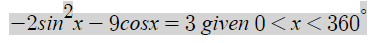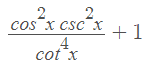# Solve for x

bigpoppapump
having trouble with the following, if anyone could provide assistance it would be appreciated.

Solve for x:and

Simplify the following:#### Attachments

jonah1
Beer soaked request follows.
having trouble with the following, if anyone could provide assistance it would be appreciated.

Solve for x:

View attachment 11112

and

Simplify the following:
View attachment 11113
Please show us what you have tried and exactly where you are stuck.

skeeter
https://mathhelpboards.com/attachments/1619175324265-png.11112/
change $\sin^2{x}$ to $(1-\cos^2{x})$ and solve the resulting quadratic equation for $\cos{x}$

https://mathhelpboards.com/attachments/1619175453718-png.11113/

change the cosecant and cotangent to factors in terms of sine & cosine, then simplify

bigpoppapump
https://mathhelpboards.com/attachments/1619175324265-png.11112/
change $\sin^2{x}$ to $(1-\cos^2{x})$ and solve the resulting quadratic equation for $\cos{x}$

https://mathhelpboards.com/attachments/1619175453718-png.11113/

change the cosecant and cotangent to factors in terms of sine & cosine, then simplify

Thank you. This helps, I was stuck but I have a good idea on how to solve both of these. Will work on it tonight.

bigpoppapump
I have managed to solved these problems with confidence which is great. Thanks for your guidance.

I have a word problem that I’m finding it difficult to convert into an equation. Could some direction be given so I can then run with it and complete.

The question is...
An electrical circuit runs at 50Hz at 0.5amps. Due to a lag in the switch, the first maximum current is reached at 6milliseconds. Assuming no variation, find an equation to model the current in this circuit using time in milliseconds.

skeeter
frequency is the reciprocal of period (time to complete one cycle of AC)

$T = \dfrac{1}{50} = 0.02 \text{ sec } = 20 \text{ milliseconds}$

current flow (with no lag) as a function of time in milliseconds ...

$A = 0.5 \sin\left(\dfrac{\pi}{10} \cdot t \right)$

For that period, the sinusoidal graph of current would peak at $\dfrac{T}{4} = 5 \text{ milliseconds}$

Due to the lag, there is a 1 millisecond horizontal shift in the graph ...